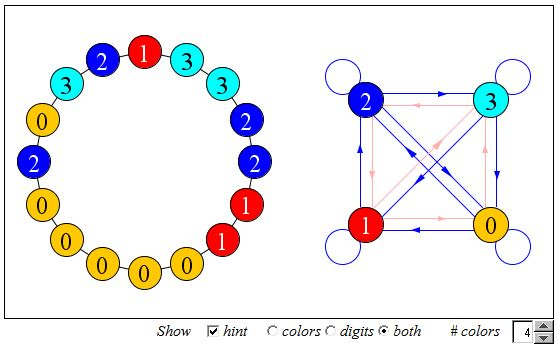# A Universal Coloring

Sixtteen dots are arranged in a circular pattern. Each can be painted with one of four colors. Paint the dots in such a way that going around the circle in either direction you will meet all possible (ordered) pairs of two colors by inspecting successive dots [Bicycle, #129].

The applet below offers you a chance to solve similar problems with the number of colors n = 2, 3, 4, 5, and 6 and the numbers of dots equal to n2. (Dots cycle through available colors when clicked upon.)

### If you are reading this, your browser is not set to run Java applets. Try IE11 or Safari and declare the site https:///www.cut-the-knot.org as trusted in the Java setup.What if applet does not run?

Discussion

### References

1. J. Konhauser, D. Velleman, S. Wagon, Which Way Did the Bicycle Go?, MAA, 1996, #129Sixtteen dots are arranged in a circular pattern. Each can be painted with one of four colors. Paint the dots in such a way that going around the circle in either direction you will meet all possible (ordered) pairs of two colors by inspecting successive dots.

### If you are reading this, your browser is not set to run Java applets. Try IE11 or Safari and declare the site https:///www.cut-the-knot.org as trusted in the Java setup.What if applet does not run?

One way to solve the problem is by relating a coloring of n2 dots with n colors to a directed graph. A directed graph, or digraph, is a combination of vertices and directed links or arcs. An arc on a digraph joins one vertex to another according to its direction which is usually indicated by an arrow. Thus it has a starting and an ending vertices. The two vertices may or may not be joined by an arc in the opposite direction. A vertex may be joined to itself, in which case the arc is known as a loop. On a digraph, each vertex has an indegree and an outdegree. The indegree equals the number of arcs that end at this vertex. In other words, the indegree of a vertex is the number of vertices joined to the given vertex. The outdegree is the number of arcs that join this vertex with other vertices. A path is a sequence of arcs in which the ending vertex of one arcs is simultaneously the starting vertex of the subsequent arc.

Let's now return to the problem of dots coloring. By fixing an orientation on the circle we make each pair of successive dots ordered. Consider a graph with n (= number of colors) vertices and paint each vertex with one of the given colors. We join two vertices with an arc iff there is an ordered pair of successive dots painted with exactly same colors. Obviously, solving the problem means finding a coloring of the dots which leads to a complete graph with n vertices, i.e. to the graph in which every vertex is joined to every other (itself, in particular) vertex.

We may also start with such a complete graph. Then a coloring of dots will correspond to a (closed) path on the graph. Within this interpretation we look for a coloring which generates an Euler cycle (also, an Eulerian circuit), i.e. a path that includes all the available arcs in some order and starts and ends at the same vertex. An Euler cycle exists on any digraph whose vertices have the property that, for each, the indegree equals the outdegree. This condition is obviously satisfied by a complete graph. Furthermore, we may start forming an Euler cycle at any vertex and proceed almost randomly: on a complete digraph there is no way to get stuck without completing the jobs!

Note that the dots coloring problem serves a particular case of de Bruijn's cycle construction.

### References

1. N. Hartsfield, G. Ringel, Pearls in Graph Theory, Dover, 1994
2. V. K. Balakrishnan, Graph Theory, Shaum's Outline Series, 1997### de Bruijn Cycles